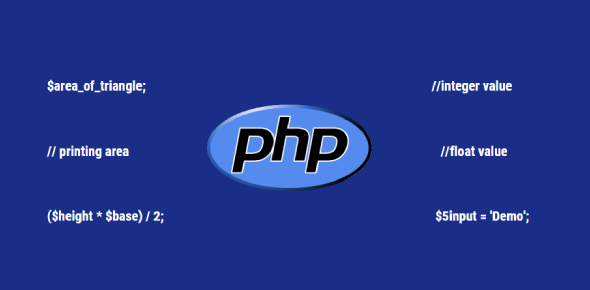# PHP Basics Variable Test

10 Questions | Attempts: 6799
ShareSettingsHave you studied PHP language before? Take this 'PHP basics variable test' that we've created below for you. PHP is a popular general-purpose scripting language that is designed to suit web development. In this quiz, we'll be asking you some general basic questions about PHP scripting language. This may also give you the chance to refresh your concepts. So, get ready and start answering. Wishing you the best of luck, buddy!

• 1.
Are the following two variables the same:\$randomString = “YouTubers”; \$randomString = “Google”;
• A.

True

• B.

False

• 2.
PHP variables are case-sensitive. True or false.
• A.

True

• B.

False

• 3.
What data type will PHP automatically convert the following variable to: \$aVariable = 99;
• A.

Integer (a number variable)

• B.

string (a text variable)

• 4.
When a variable is declared, it can only be used 1 time in the PHP source file. State true or false?
• A.

True

• B.

False

• 5.
All variables in PHP start with a \$(dollar) sign. True or false?
• A.

True

• B.

False

• 6.
What data type will PHP automatically convert the following variable to: \$aVariable = “Robert”;
• A.

Integer (a number variable)

• B.

String (a text variable)

• 7.
PHP variables should be declared before adding a value to it.
• A.

True

• B.

False

• 8.
Which of the following options represents the incorrect way to declare a PHP variable?
• A.

\$aVariable;

• B.

\$a_Variable = 9;

• C.

\$AVariable;

• D.

\$a Variable;

• 9.
Which of the following is the incorrect way to declare a PHP variable?
• A.

\$a_Number = 9;

• B.

\$a_Number = 9

• C.

\$a_Num = 9;

• D.

\$aNumber = 9;

• 10.
Are the following two variables the same: \$yValue = 7; \$YValue = 7;
• A.

True

• B.

False

## Related TopicsBack to top
×

Wait!
Here's an interesting quiz for you.